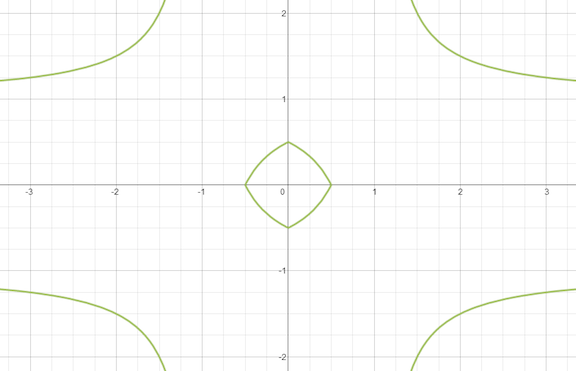# Pillowed Diamond

Calculus Level 2Above is the graph of the equation $|x|+|y|-|x||y|=0.5$ Let the area of the cute little pillowed diamond in the middle be $A$. What is the value of $\lfloor 1000A\rfloor$?

×